# Theory of interest

Associate Professor in Economics at Far West University of Nepal en Tikapur Multiple Campus
20 de Sep de 2020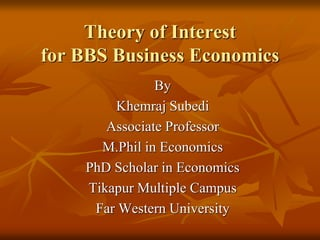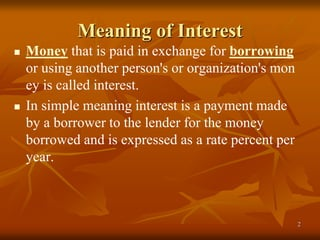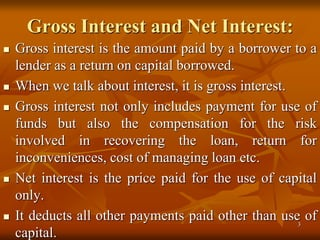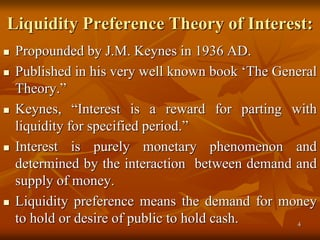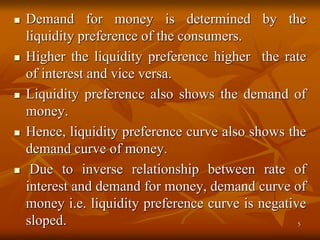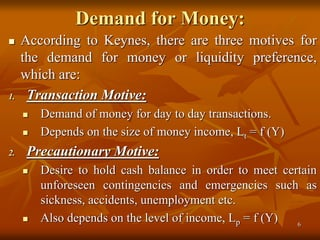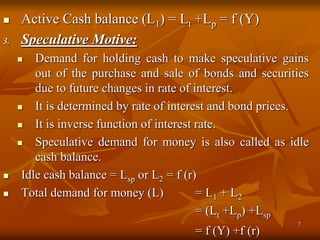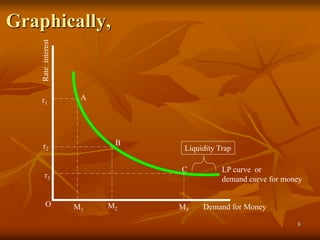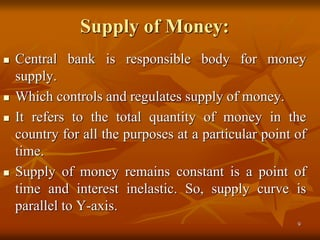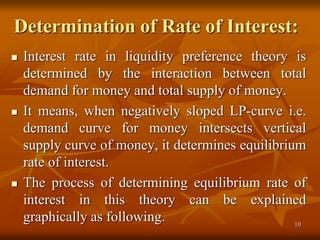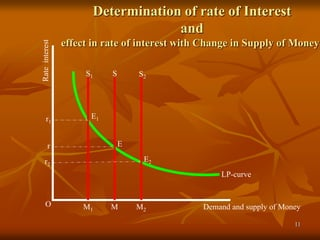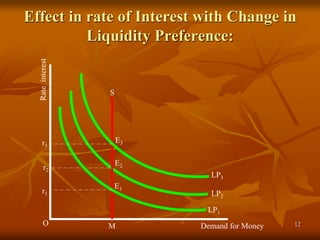1 de 13

### Theory of interest

1. Theory of Interest for BBS Business Economics By Khemraj Subedi Associate Professor M.Phil in Economics PhD Scholar in Economics Tikapur Multiple Campus Far Western University
2. Meaning of Interest  Money that is paid in exchange for borrowing or using another person's or organization's mon ey is called interest.  In simple meaning interest is a payment made by a borrower to the lender for the money borrowed and is expressed as a rate percent per year. 2
3. Gross Interest and Net Interest:  Gross interest is the amount paid by a borrower to a lender as a return on capital borrowed.  When we talk about interest, it is gross interest.  Gross interest not only includes payment for use of funds but also the compensation for the risk involved in recovering the loan, return for inconveniences, cost of managing loan etc.  Net interest is the price paid for the use of capital only.  It deducts all other payments paid other than use of capital. 3
4. Liquidity Preference Theory of Interest:  Propounded by J.M. Keynes in 1936 AD.  Published in his very well known book ‘The General Theory.”  Keynes, “Interest is a reward for parting with liquidity for specified period.”  Interest is purely monetary phenomenon and determined by the interaction between demand and supply of money.  Liquidity preference means the demand for money to hold or desire of public to hold cash. 4
5.  Demand for money is determined by the liquidity preference of the consumers.  Higher the liquidity preference higher the rate of interest and vice versa.  Liquidity preference also shows the demand of money.  Hence, liquidity preference curve also shows the demand curve of money.  Due to inverse relationship between rate of interest and demand for money, demand curve of money i.e. liquidity preference curve is negative sloped. 5
6. Demand for Money:  According to Keynes, there are three motives for the demand for money or liquidity preference, which are: 1. Transaction Motive:  Demand of money for day to day transactions.  Depends on the size of money income, Lt = f (Y) 2. Precautionary Motive:  Desire to hold cash balance in order to meet certain unforeseen contingencies and emergencies such as sickness, accidents, unemployment etc.  Also depends on the level of income, Lp = f (Y) 6
7.  Active Cash balance (L1) = Lt +Lp = f (Y) 3. Speculative Motive:  Demand for holding cash to make speculative gains out of the purchase and sale of bonds and securities due to future changes in rate of interest.  It is determined by rate of interest and bond prices.  It is inverse function of interest rate.  Speculative demand for money is also called as idle cash balance.  Idle cash balance = Lsp or L2 = f (r)  Total demand for money (L) = L1 + L2 = (Lt +Lp) +Lsp = f (Y) +f (r) 7
8. Graphically, O Demand for Money Rateinterest LP curve or demand curve for money A B C Liquidity Trap r1 r2 r3 M1 M2 M3 8
9. Supply of Money:  Central bank is responsible body for money supply.  Which controls and regulates supply of money.  It refers to the total quantity of money in the country for all the purposes at a particular point of time.  Supply of money remains constant is a point of time and interest inelastic. So, supply curve is parallel to Y-axis. 9
10. Determination of Rate of Interest:  Interest rate in liquidity preference theory is determined by the interaction between total demand for money and total supply of money.  It means, when negatively sloped LP-curve i.e. demand curve for money intersects vertical supply curve of money, it determines equilibrium rate of interest.  The process of determining equilibrium rate of interest in this theory can be explained graphically as following. 10
11. O Demand and supply of Money Rateinterest r LP-curve S M S1 S2 E E1 E2 M1 M2 r1 r2 Determination of rate of Interest and effect in rate of interest with Change in Supply of Money: 11
12. Effect in rate of Interest with Change in Liquidity Preference: O Demand for Money Rateinterest r2 LP2 S M E2 LP3 LP1 r1 r3 E1 E3 12
13. Thank You! 13